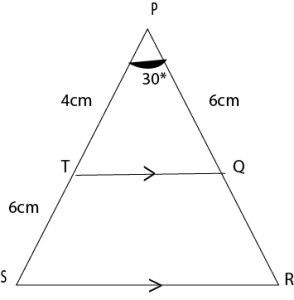# Mathematics 2018 Past Questions (Theory) | WAEC

Study the following Mathematics past questions and answers for JAMBWAEC  NECO and Post-JAMB. Get prepared with official past questions and answers for upcoming examinations.

## Theory

1. A used car was purchased at N900,000.00. Its value depreciated by 30% in the first year. In each subsequent year, the depreciation was 22% of its value at the beginning of the year. If the car was bought on the 1st of March, 2011, calculate, correct to the nearest hundred naira, the value of the car on the 28th of February, 2015.
${}^{}$

2. (a) The graph of

y=2px14

passes through the point (3, 10). Find the values of p.

(b) Two lines,

$3y-2x=21$

and  $4y+5x=5$

intersect at the point Q. Find the coordinates of Q.

3. (a) The diagonals of a rhombus are 10.2 cm and 9.3 cm long. Calculate, correct to one decimal place, the perimeter of the rhombus.

(b) Given that sinx=3/5, 0°< x <90°, find the value of 5cosx4tanx

4.

(a)In the diagram

(i) The value of x; (ii) <RSQ

(b) If  2N4seven=15Nnine,

find the value of N.

5. (a) If the mean of m, n, s, p and q is 12, calculate the mean of (m + 4), (n – 3), (s + 6), (p – 2) and (q + 8).

(b) In a community of 500 people, the 75th percentile age is 65 years while the 25th percentile age is 15 years. How many of the people are between 15 and 65 years?

6. In a road worthiness test on 240 cars, 60% passed. The number that failed had faults in Clutch, Brakes and Steering as follows: Clutch only – 28, Clutch and Steering – 14; Clutch, Steering and Brakes – 8; Clutch and Brakes – 20; Brakes and Steering only – 6. The number of cars with faults in Steering only is twice the number of cars with faults in Brakes only.

(a) Draw a Venn Diagram to illustrate this information.

(b) How many cars had : (i) Faulty Brakes?  (ii)  Only one fault?

7. (a) Find the equation of the line passing through the points (2, 5) and (-4, -7).

(b) Three ships P, Q and R are at sea. The bearing of Q from P is 030° and the bearing of P and R is 300°.  If |PQ| = 5 km and |PR| = 8 km,

(i) Illustrate the information in a diagram.

(ii) Calculate, correct to three significant figures, the:

(1) distance between Q and R

(2) bearing of R from Q.

8. (a) Lamin bought a book for N300.00 and sold it to Bola at a profit of x%. Bola then sold the same book at a profit of x%. If James paid

$N\left(6x+\frac{3}{4}\right)$

more for the book than Lamin paid, find the value of x.

(b) Find the range of values of x which satisfies the inequality

$3x-2<10+x<2+5x$

.

9.In the diagram, |PT| = 4 cm, |TS| = 6 cm, |PQ| = 6 cm and < SPR = 30°. Calculate, correct to the nearest whole number:

(a) |SR| ;

(b) area of TQRS.

10.90°, Find, correct to three significant figures, |PR|.

(b) The length of two ladders, L and M are 10m and 12m respectively. They are placed against a wall such that each ladder makes angle with the horizontal ground. If the foot of L is 8m from the foot of the wall.

(i) Draw a diagram to illustrate this information;  (ii) Calculate the height at which M touches the wall.

11. (a) Copy and complete the table of values for y=2+x10 for 5x4

 x -5 -4 -3 -2 -1 0 1 2 3 4 y 5 -9 -10 0

(b) Using scales of 2cm to 1 unit on the x- axis and 2cm to 5 units on the y- axis, Draw the graph of y=2+x1 for 5x4.

(c) Use the graph to find the solution of :

(i) 2+x=10

(ii) 2+x10=2x

12.

 Marks 10 20 30 40 50 60 70 80 90 Frequency 1 1 x 5 y 1 4 3 1

The frequency distribution shows the marks distribution of a class of 30 students in an examination.

The mean mark of the distribution is 52.

(a) Find the values of x and y.

(b) Construct a group frequency distribution table starting with a lower class limit of 1 and class interval of 10.

(c) Draw a histogram for the distribution

(d) Use the histogram to estimate the mode

## For Enquiries?

Scroll to Top